You are currently offline. Some features of the site may not work correctly.

# Minimal polynomial (linear algebra)

Known as: Algebraic number minimal polynomial, Minimum polynomial, Existence of the minimal polynomial
In linear algebra, the minimal polynomial μA of an n × n matrix A over a field F is the monic polynomial P over F of least degree such that P(A) = 0… Expand
Wikipedia

## Papers overview

Semantic Scholar uses AI to extract papers important to this topic.
Highly Cited
2006
Highly Cited
2006
• SIAM J. Sci. Comput.
• 2006
• Corpus ID: 1200662
We consider the application of the conjugate gradient method to the solution of large, symmetric indefinite linear systems… Expand
•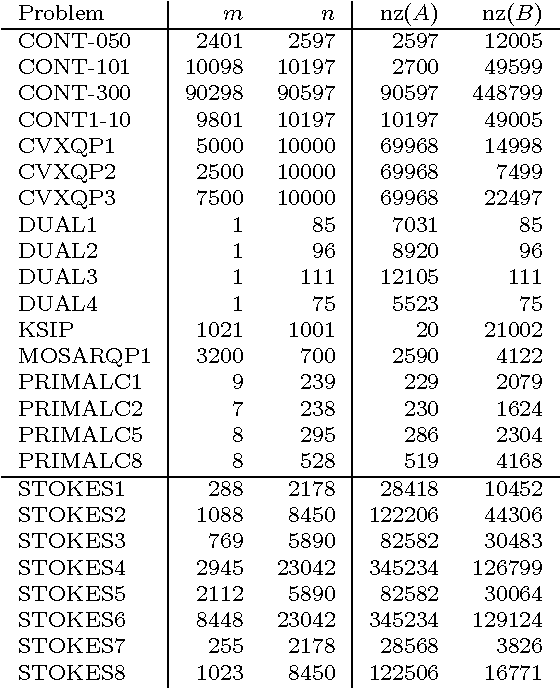•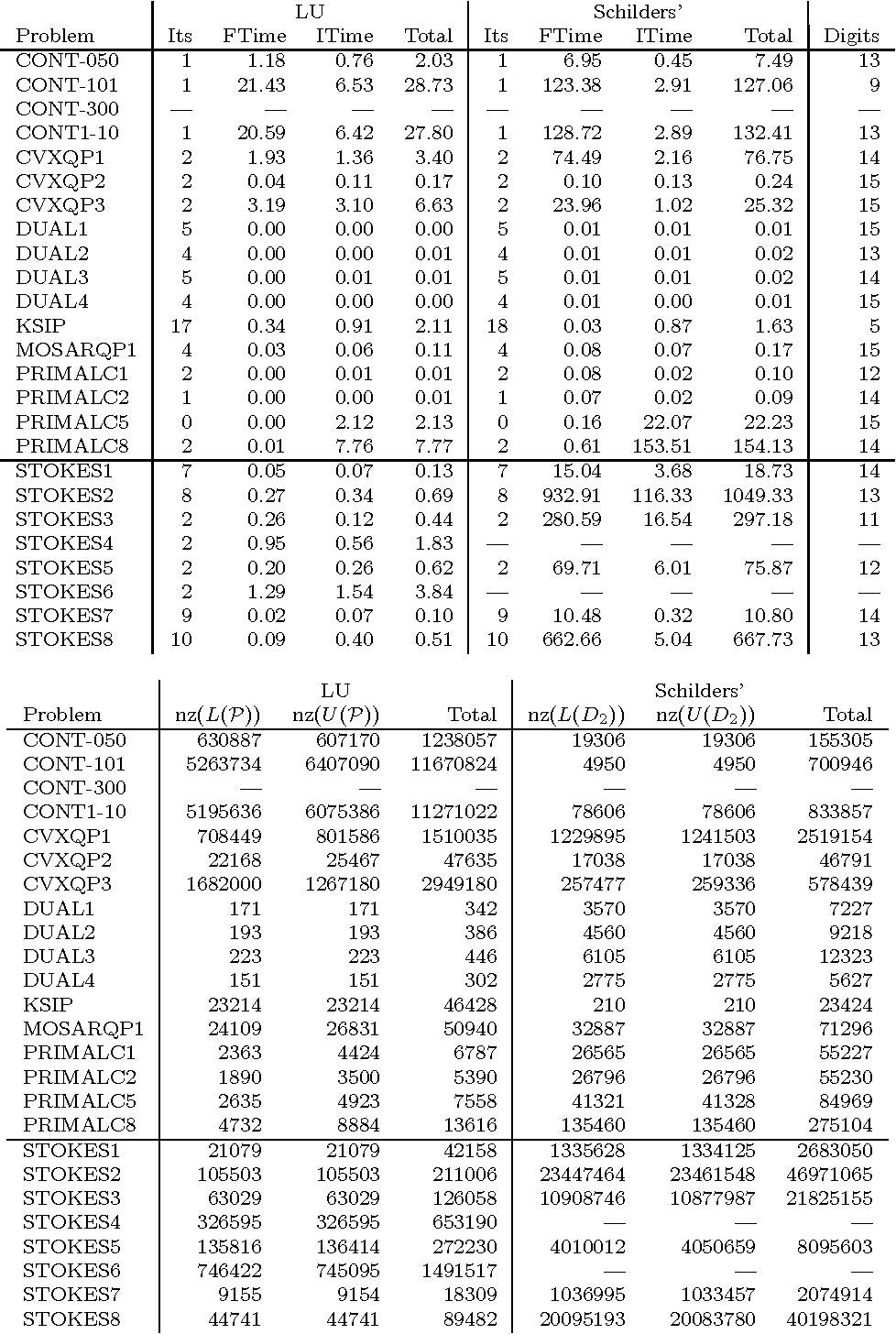•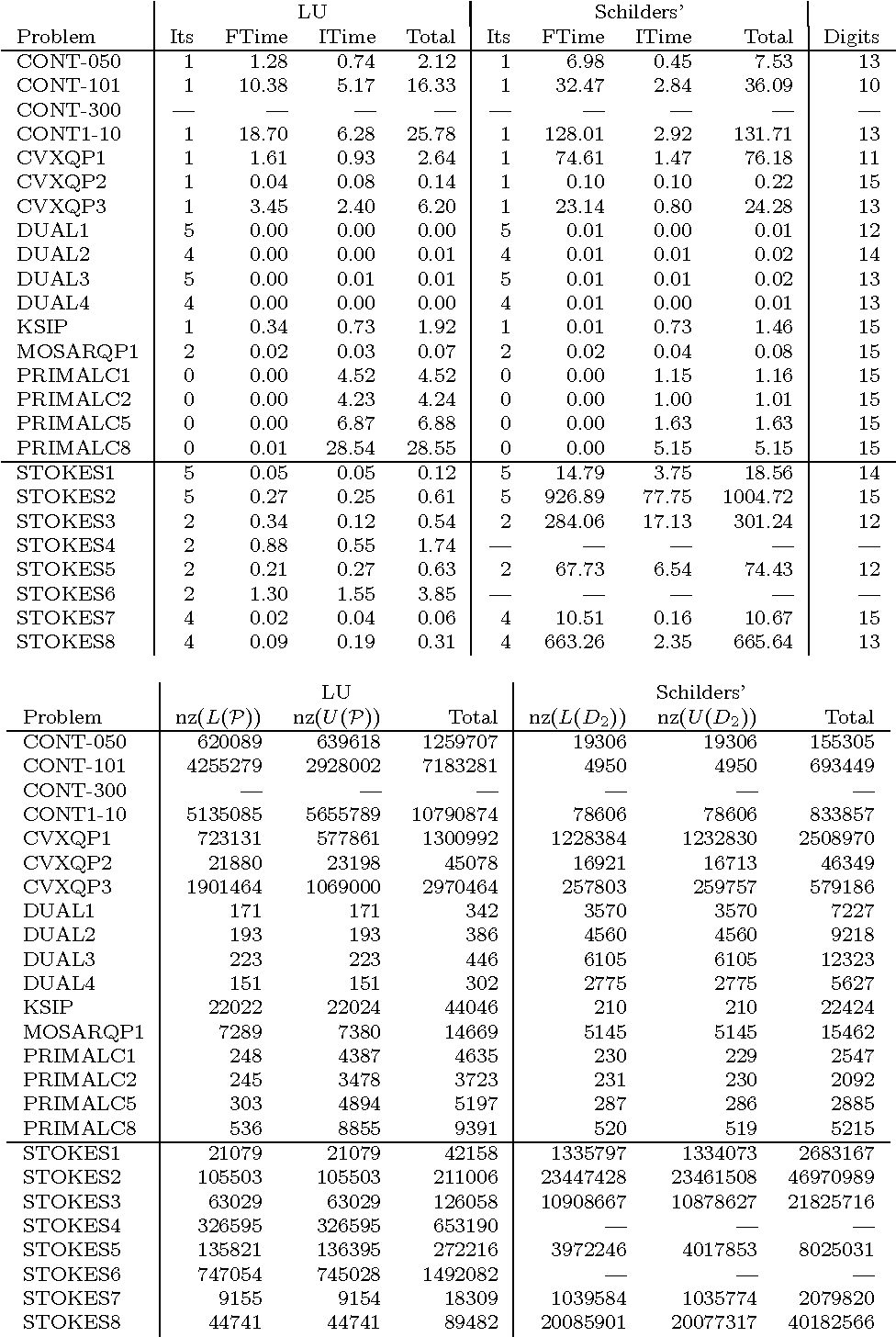•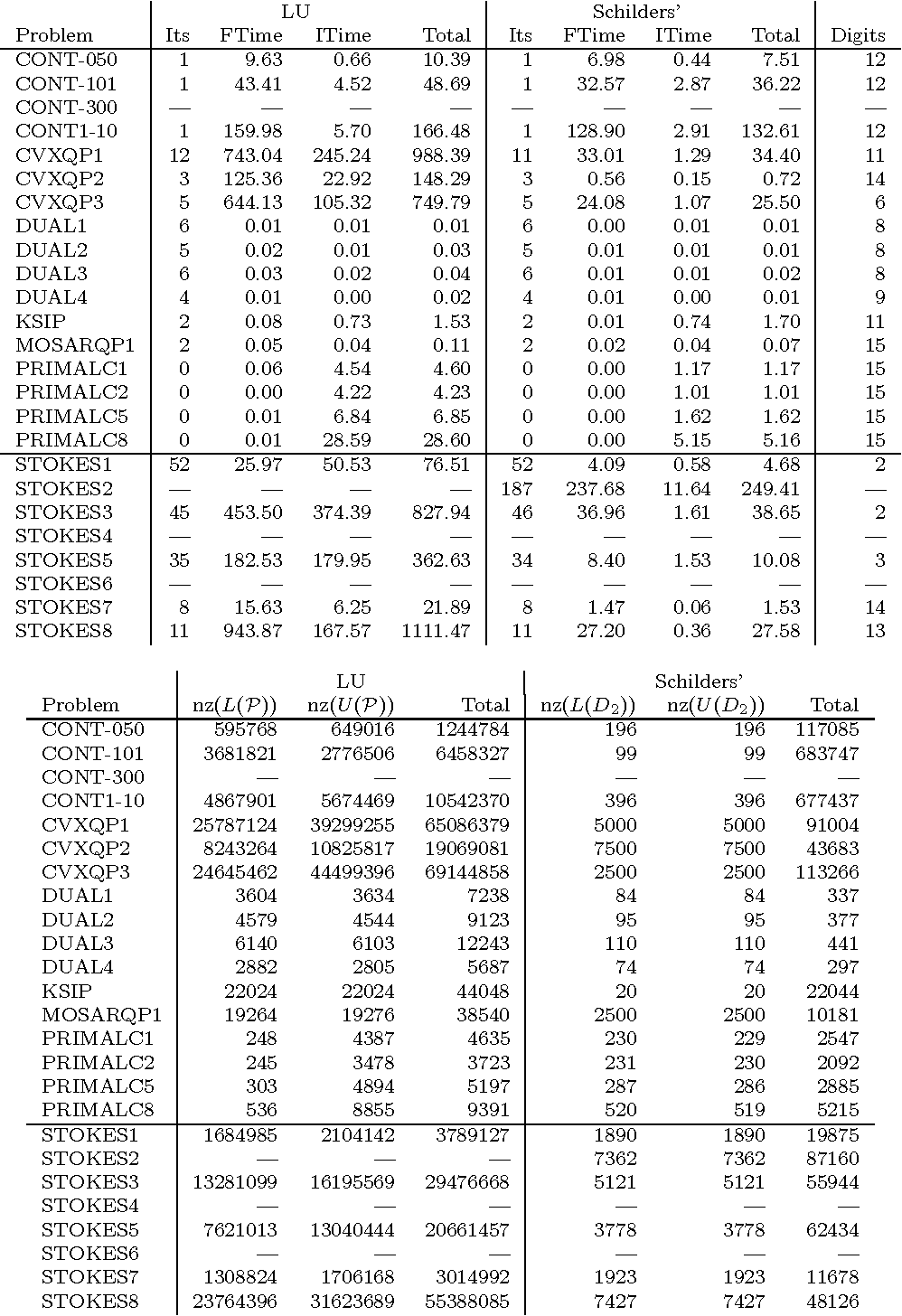•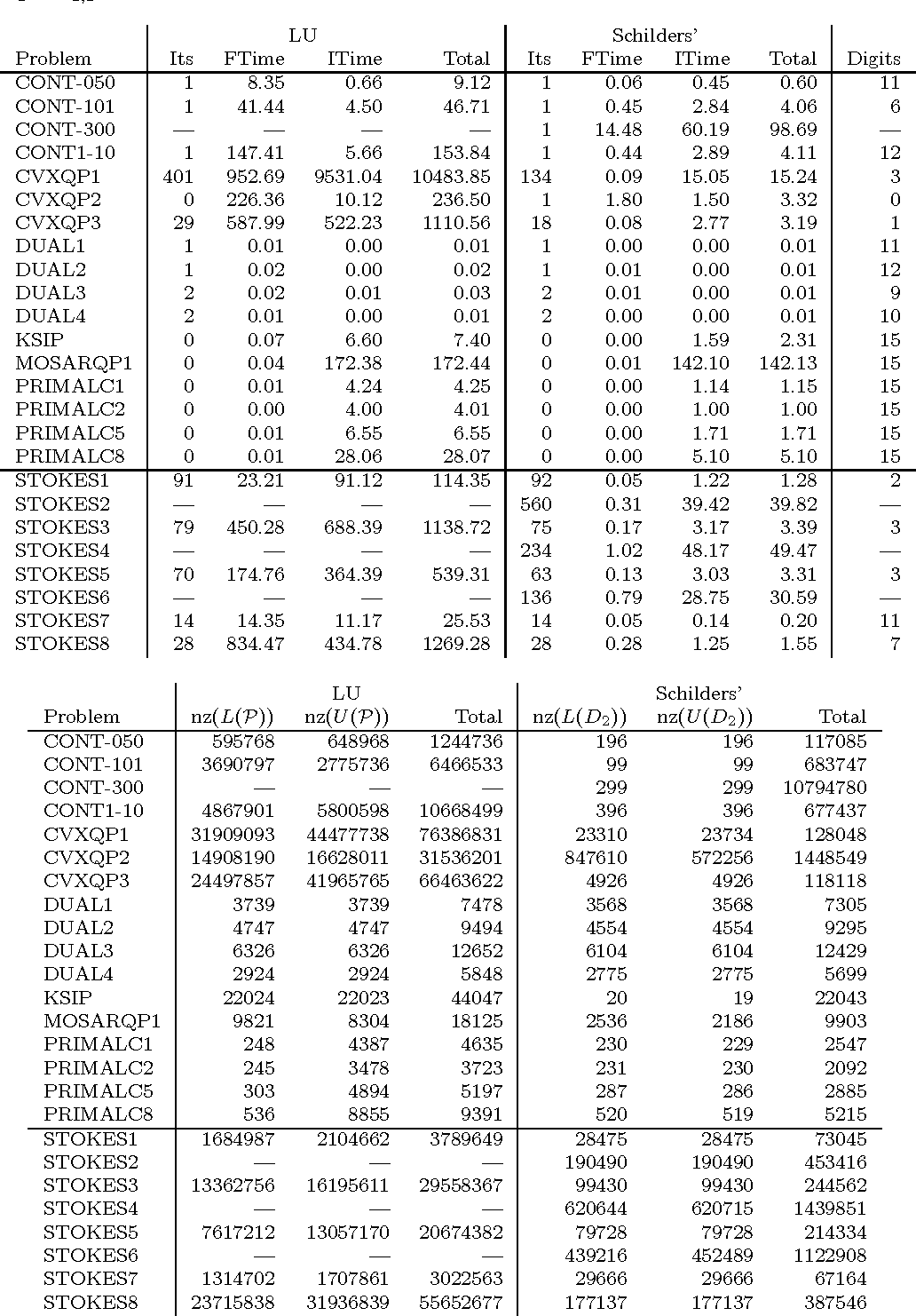Is this relevant?
2000
2000
• Math. Comput.
• 2000
• Corpus ID: 464224
In this paper we analyze the bi-conjugate gradient algorithm in finite precision arithmetic, and suggest reasons for its often… Expand
•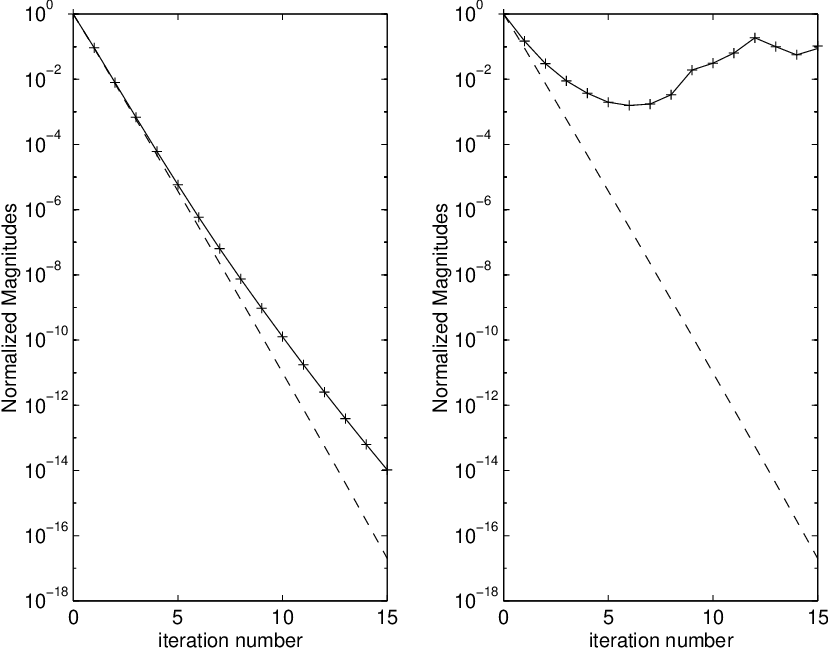•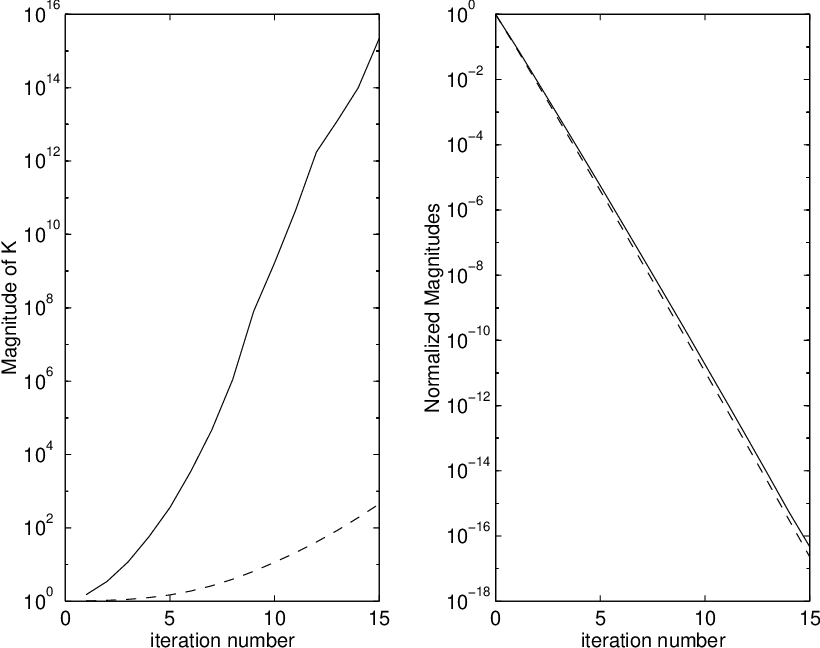•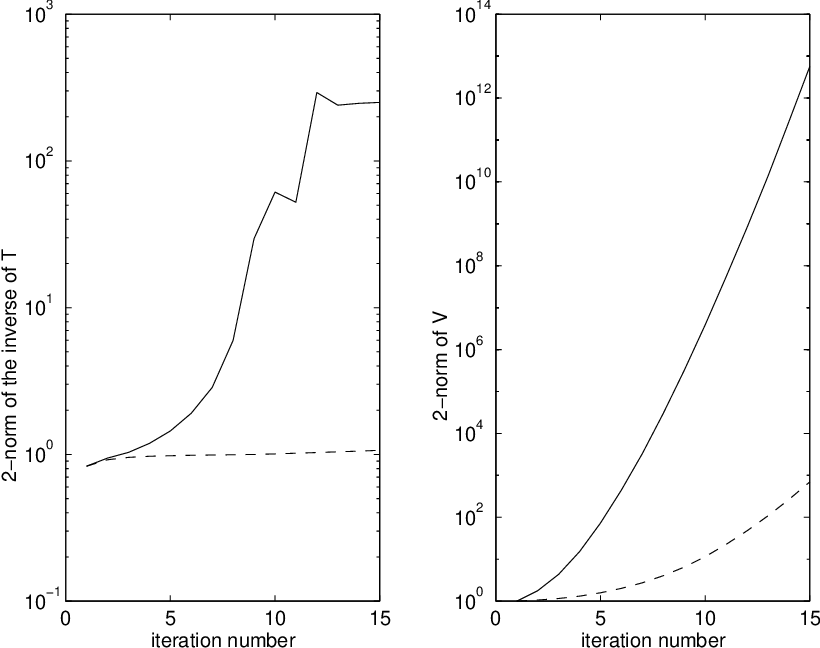•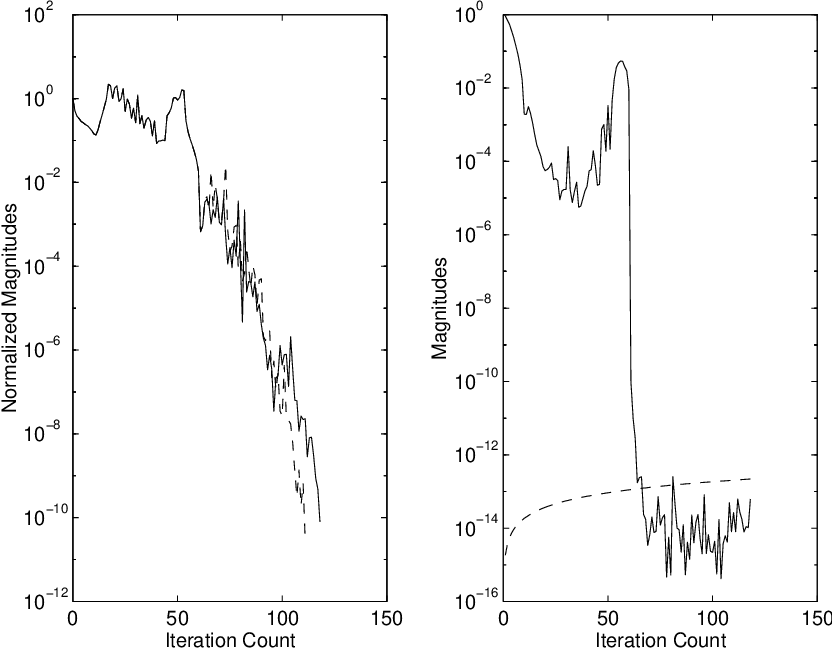Is this relevant?
Highly Cited
2000
Highly Cited
2000
• SIAM J. Matrix Anal. Appl.
• 2000
• Corpus ID: 34087888
The problem of finding good preconditioners for the numerical solution of indefinite linear systems is considered. Special… Expand
•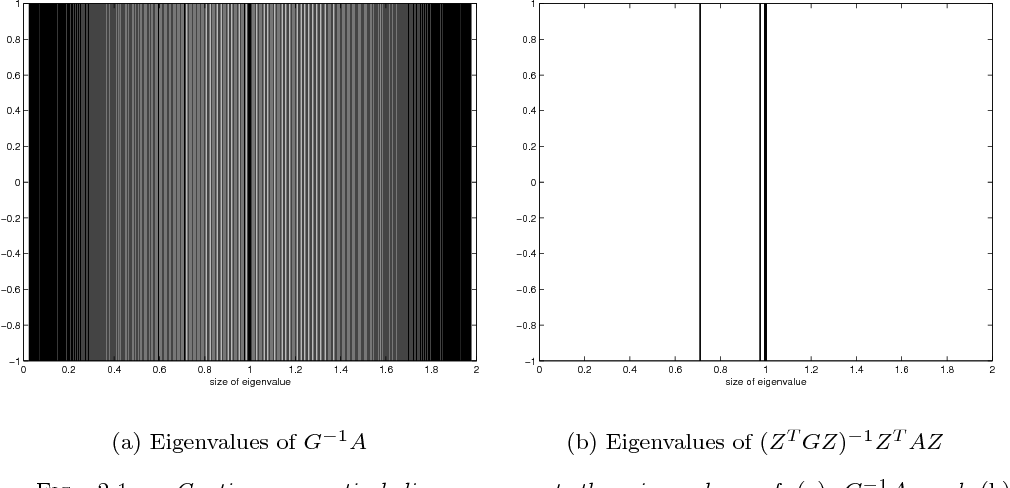•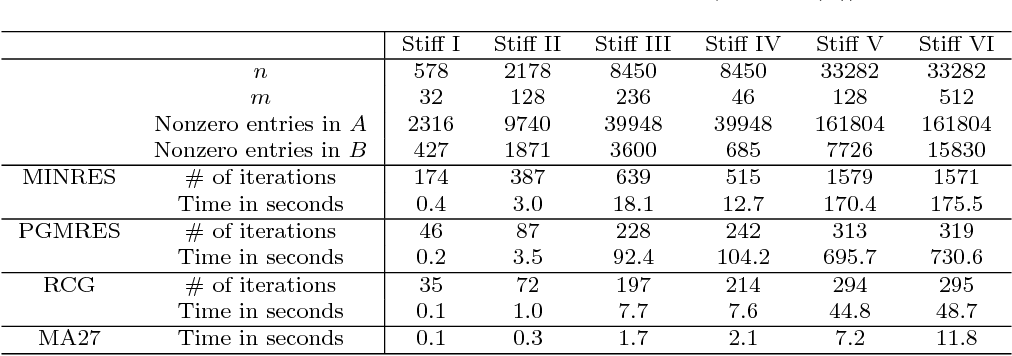•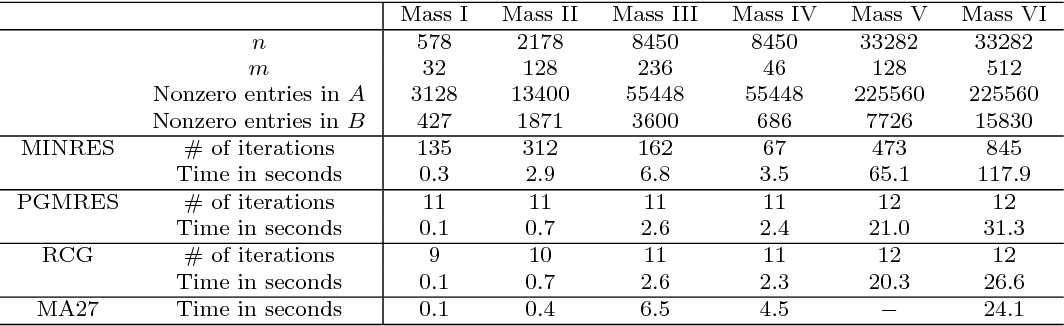•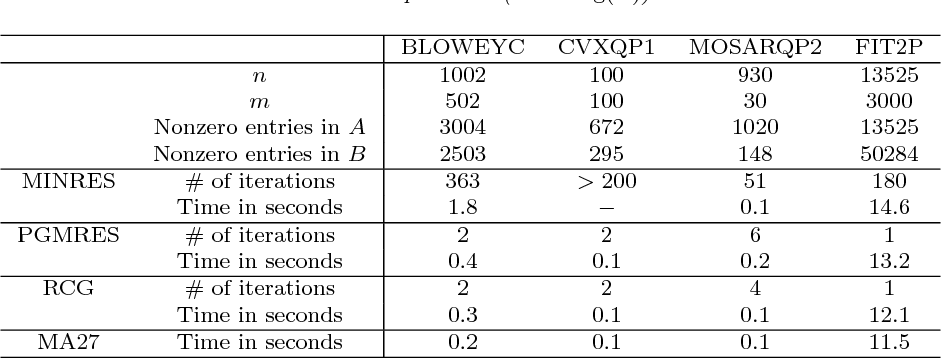Is this relevant?
Highly Cited
1996
Highly Cited
1996
• 1996
• Corpus ID: 2713035
We present a qualitative model for the convergence behaviour of the Generalised Minimal Residual (GMRES) method for solving… Expand
Is this relevant?
1995
1995
In this paper we prove the following theorem: if the Riccati equation w? + w2 = R(x), R ? Q(x), has algebraic solutions, then… Expand
Is this relevant?
1991
1991
• 1991
• Corpus ID: 8778629
A procedure for the identification of industrial processes with the intention of control system design is proposed, discussed… Expand
•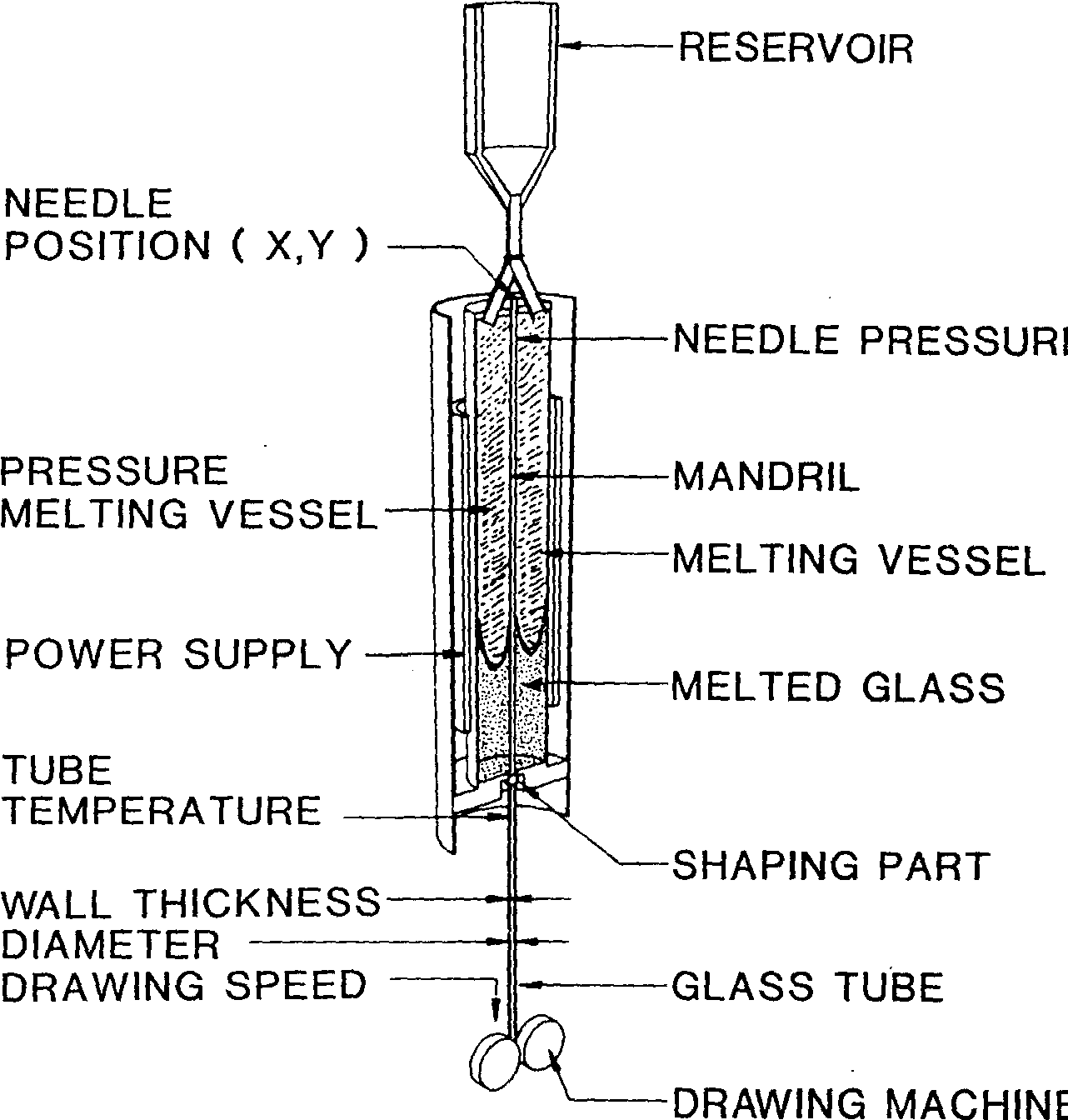•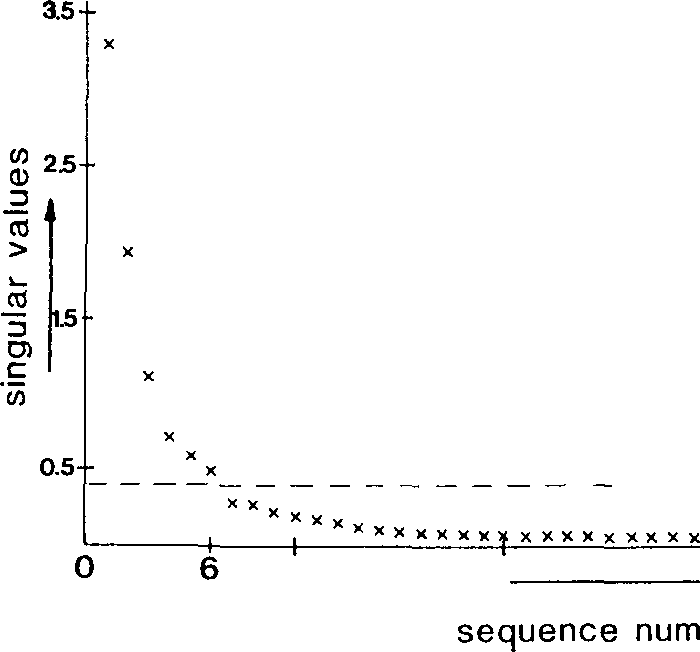•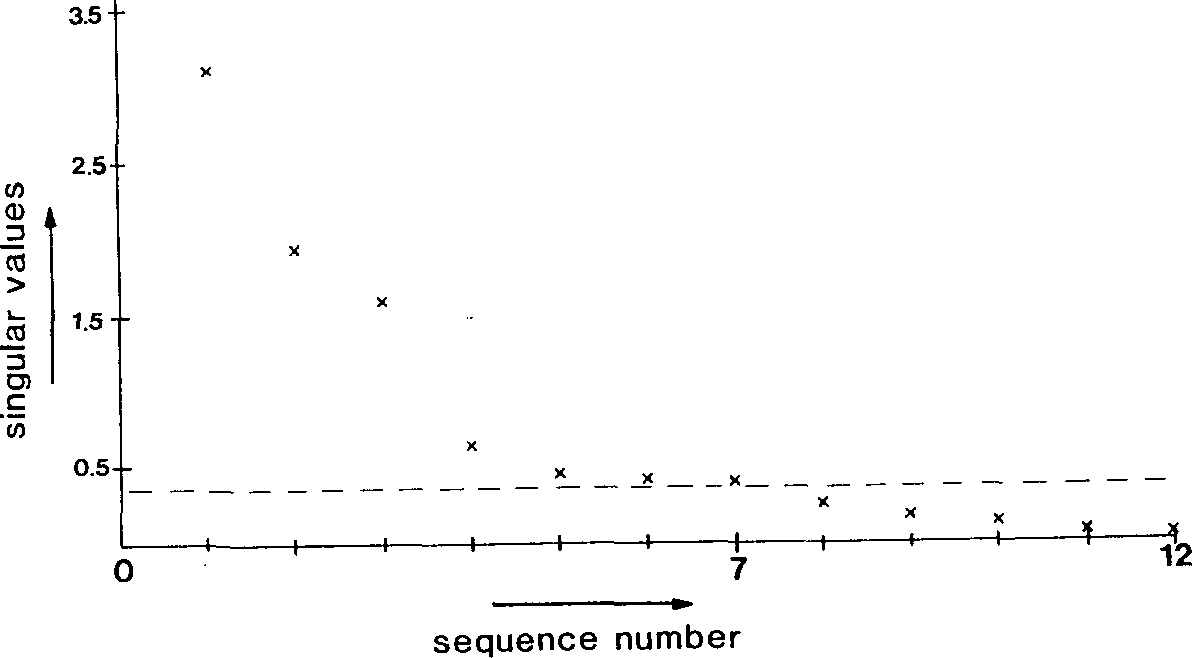•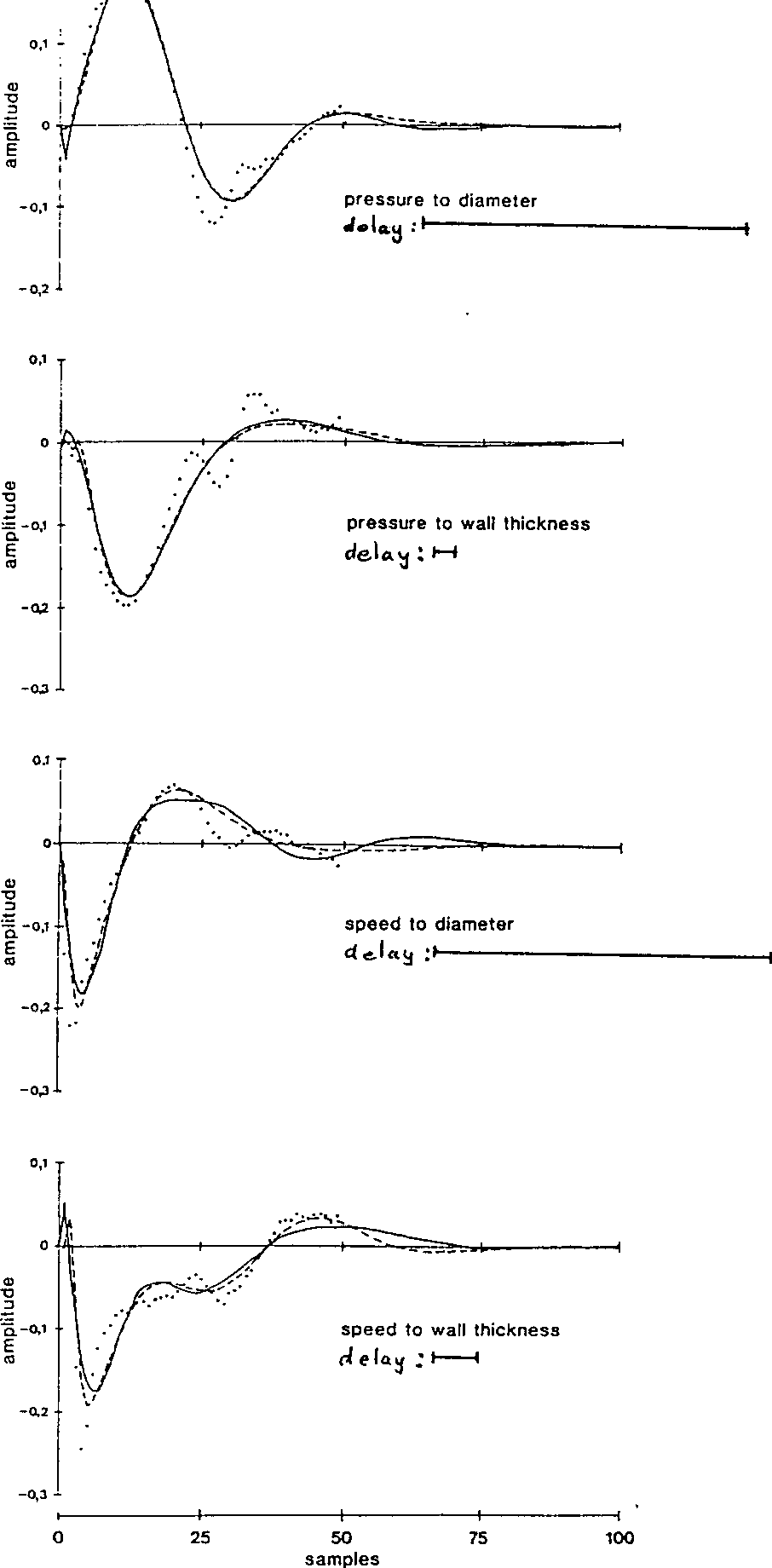•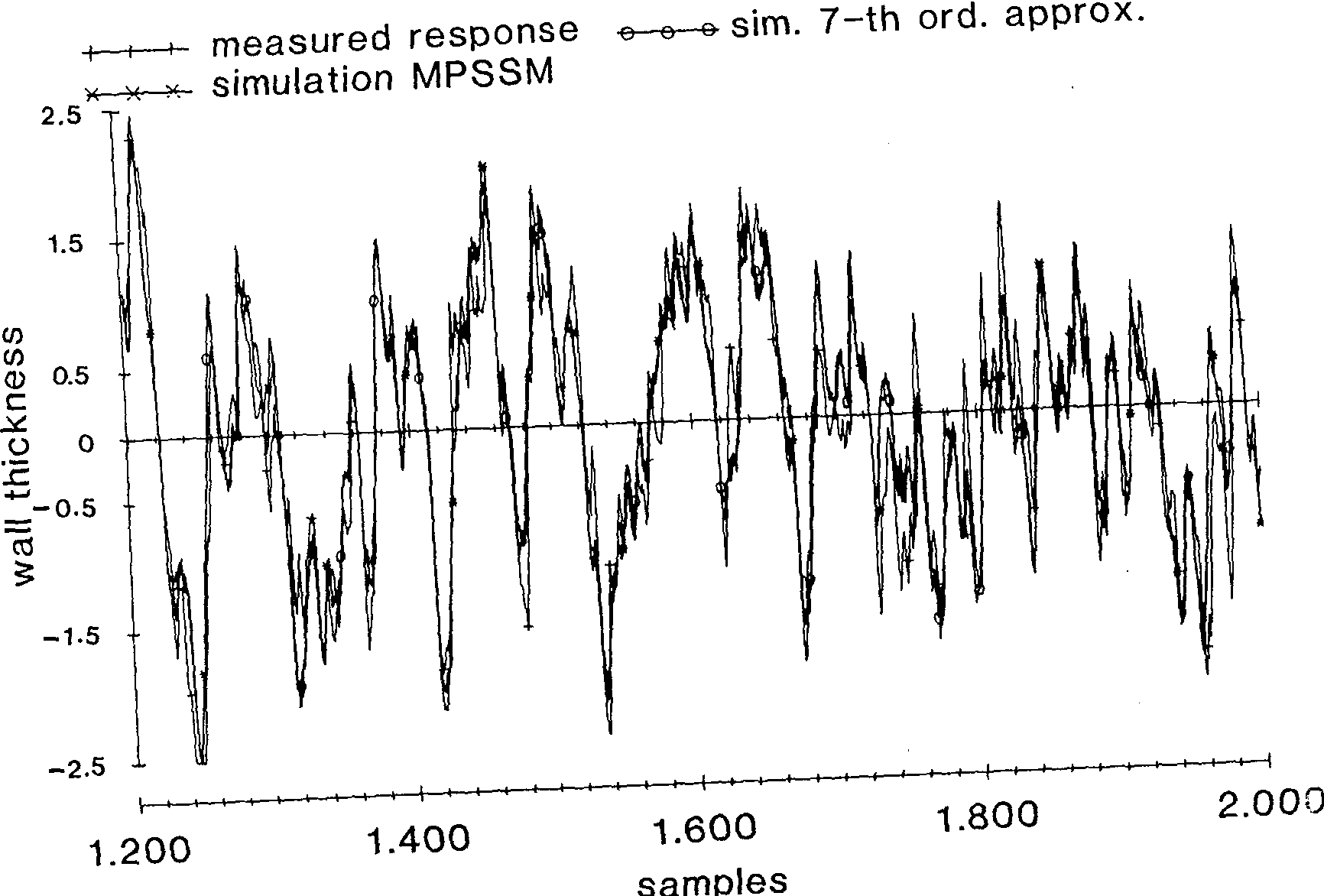Is this relevant?
Highly Cited
1987
Highly Cited
1987
Introduction to the Theory of Boolean Functions and Circuits. The Minimimization of Boolean Functions. The Design of Efficient… Expand
•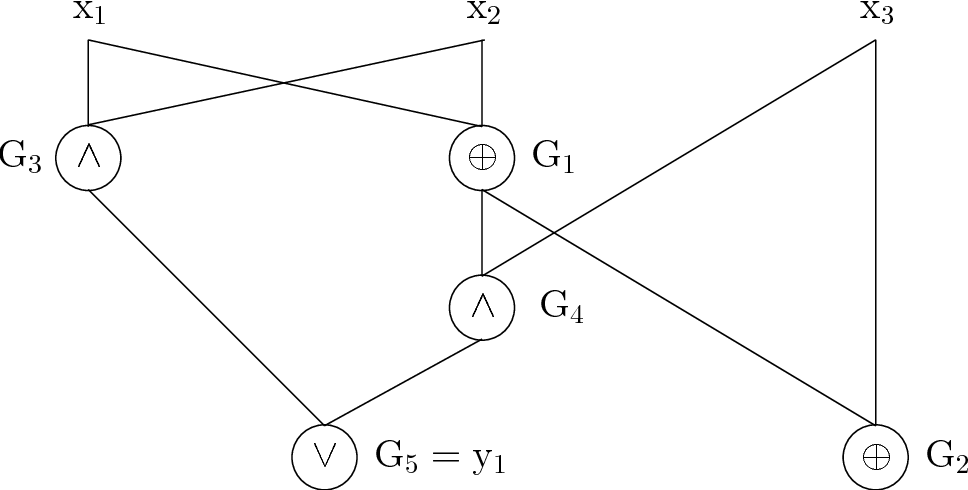•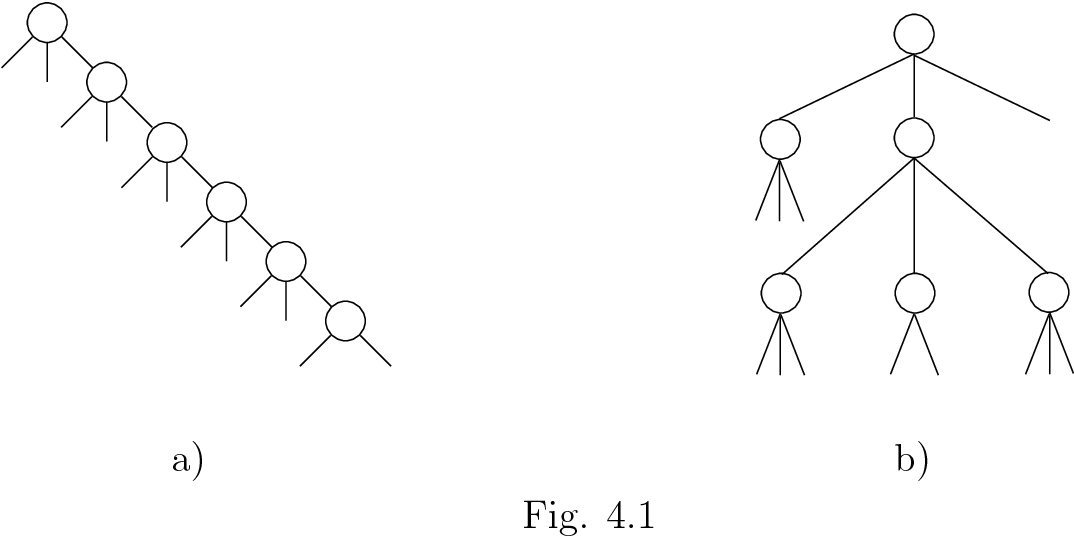•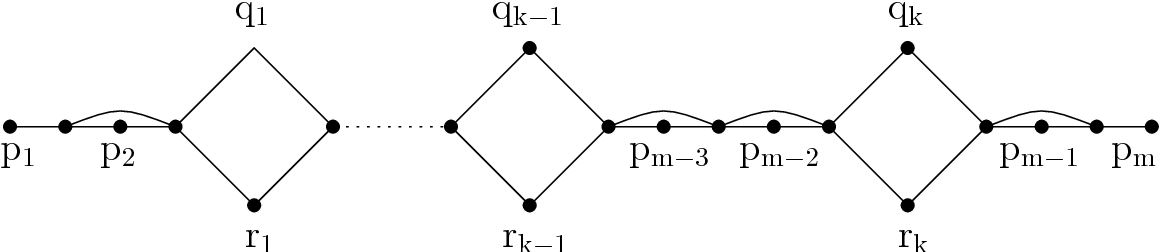•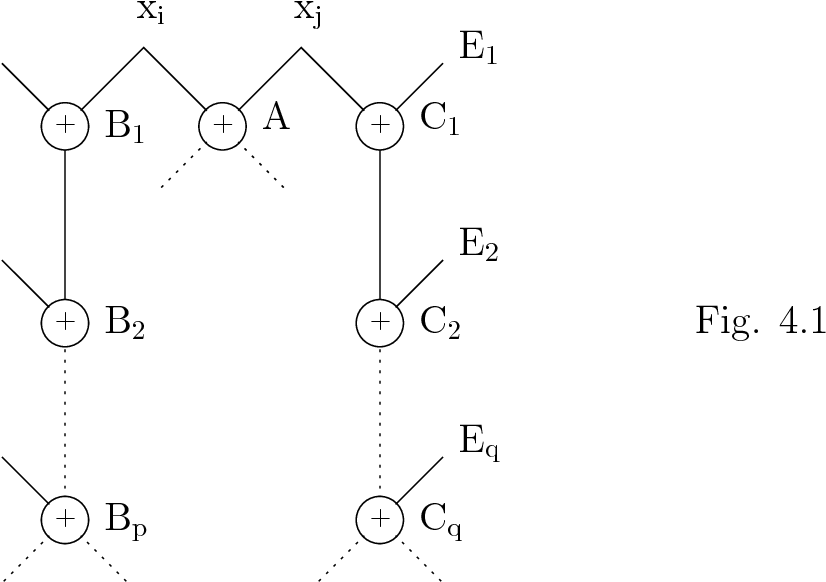•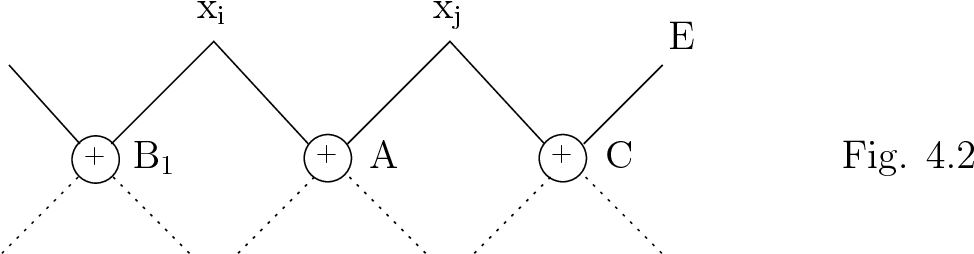Is this relevant?
Highly Cited
1986
Highly Cited
1986
A "coordinate recurrence" method for solving sparse systems of linear equations over finite fields is described. The algorithms… Expand
Is this relevant?
1982
1982
In recent years considerable interest has been shown in the generation of binary sequences which have good randomness properties… Expand
Is this relevant?
Highly Cited
1967
Highly Cited
1967
In this paper we study finite transitive groups G acting on a set Q. The results, which are trivial for multiply-transitive… Expand
Is this relevant?